# Algorithm Analysis

Tom Kelliher, CS23

Apr. 21, 1996

Algorithm analysis covers:

• Running time (worst, average case)
• Correctness

What determines running time?

• Hardware.
• The input.
• Software (system and user).

Questions:

• How is it fair to compare two programs?

• Should we compare?

• What should we compare?

• Why should we compare?

# Execution Time

• Can we measure time?

• What do we measure?

• Measure as a function of what?

• Idea: count something.

## Examples

What should we count?

• Sorting employee records.

• Searching employee records.

• Finding a path in a graph.

• Eight queens problem.

# Counting

How many times does the inner loop body execute?

```for (i = 0; i < n; ++i)
for (j = 0; j < i; ++j)
; // inner loop body
```

Find closed forms for: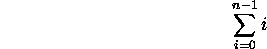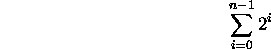# Growth Rates

• Constant.
• Logarithmic. (Familiarity with properties of logarithms?)
• Linear.
• Polynomial.
• Exponential.
Examples of problems that grow at these rates?

# The Order of an Algorithm

Definition: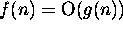if constants c and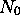exist such thatfor all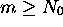.

Consequences:

• Ignore low order terms.
• Ignore multiplicative constants.
• Big Oh is distributive.

Examples:

1.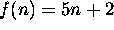2.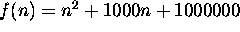A fallacious example: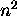is O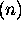.

Another:is O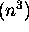.

Typical growth rates (from slowest to fastest)

Worst-case analysis.

Average-case analysis.

# Perspective

Why use a difficult, subtle, fast algorithm on small inputs?

# Application

What is the efficiency of

• Bubble sort.
• Merge sort.
• Linear search.
• Binary search.

# Exercises

Implement merge sort (don't look in the textbook!).

Thomas P. Kelliher
Sat Apr 19 13:42:29 EDT 1997
Tom Kelliher